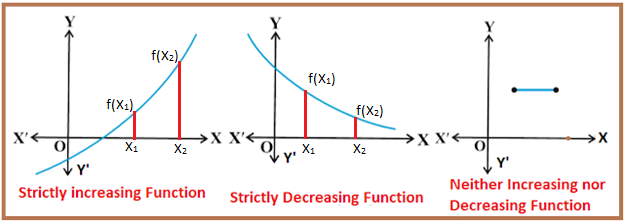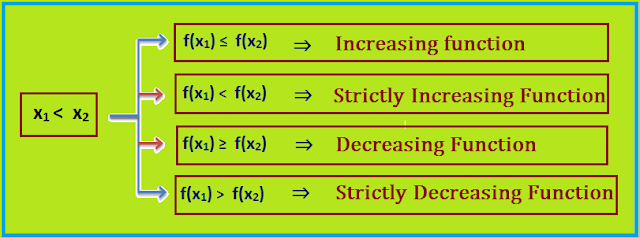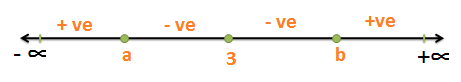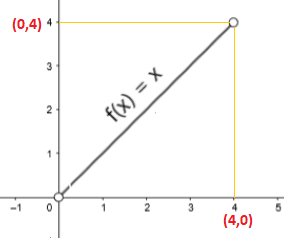### Common Errors in Secondary Mathematics

Common Errors Committed  by the  Students  in Secondary Mathematics   Errors  that students often make in doing secondary mathematics  during their practice and during the examinations  and their remedial measures are well explained here stp by step.  Some Common Errors in Mathematics

### Application of Derivatives Class XII Chapter 6

Application of Derivatives Class XII Chapter 6
Lear basic concepts of math on chapter 6 class XII, concept of slope of tangent and normal, equation of tangent and normal, concept of increasing and decreasing of a function, concept of maximum and minimum value, method of approximation

In this Chapter we study the following concepts

1) Rate of change of quantities.
2) Increasing and decreasing of the functions.
3) Equation of Tangent and equation of Normal.
4) Method of approximation.
5) Maximum and Minimum values.

1) Rate of change of quantities
Algorithm
i) Write y = f(x)
ii) Find the derivative of y with respect to other variable.
iii) Find the value of the derivative according to the question.
Note:
dy/dx means that rate of change of y with x
dS/dt means rate of change of distance with time t.
dA/dt means rate of change of Area with time.

## Concept of increasing and decreasing and their graphical representation.

(i) Increasing on I if x1 < x2  on I

(ii) Strictly increasing on I if x< x2  on I

(iii) Decreasing on I if x< x2  on I

(iv)  Strictly Decreasing on I if x< x2  on IConclusionsCritical Points :
* The values of x for which f '(x) = 0 or not differentiable are known as critical points.
* All those points at which f(x) or f ' (x) are continuous but not differentiable are called critical points.
* These are the points at which the given function is either zero or become undefined.

Method of finding Strictly Increasing and Strictly Decreasing of the function
Algorithm
a) Write the given function as f(x)
b) Differentiate this function with respect to x to find f '(x)
c) Find the critical points by putting f '(x) = 0
d) Use baby curve method to find the sign (+ ve or - ve) for f '(x)

RESULTS

1) f (x) is strictly increasing in (a, b), if f '(x) > 0 for each x ϵ (a, b)
2) f (x) is increasing in [a, b], If f '(x) ≥ 0 for each x ϵ (a, b)
3) f (x) is strictly decreasing in (a, b) if f '(x) < 0 for each x ϵ (a, b)
4) f (x) is decreasing in [a, b] If f'(x) ≤ 0 for each x ϵ (a, b)
5) If f '(x) = 0 for each x ϵ (a, b), then f (x) is a constant function in [a, b] and the constant function is neither increasing nor decreasing.

 Use of Baby Curve Method in increasing and decreasing of functionsSteps to be followed: Let given function is f(x) Find f '(x) and make the coefficient of x positive. For finding critical points put f ' (x) = 0 and find the values of variable  say x Let the values of x are a, b, c Now draw a real number line from -∞ to +∞ Place all the critical points a, b, c on the number line. Start from the extreme right hand side and place +ve sign in the interval (c, ∞) Place - ve sign, to the next interval i.e. in (b, c). Place + ve sign, to the next interval i.e. in (a, b). Place - ve sign, to the next interval i.e. in (- ∞, c). Note: We cannot change the sign at the critical point whose factor is with the even power. Example: If any factor of f '(x) is  (x - 2)2 or (x - 3)4 , then we cannot change the sign on the baby curve at point 2 and 3. Here we maintain the same sign .Power of (x - 3) is an even number so here sign remain unchanged.

Note:
Domain of exponential functions is : R (All real numbers)
Domain of log functions : (0, ∞ ) and Log x is undefined at x = 0

## Equation of Tangent and Equation of Normal to the Curve

Tangent to a curve:

It is a line which just touches the curve at one point.

Normal line:

At every tangent point there is a line which is perpendicular to the tangent line is called a normal line.

To find the equation of tangent we should have slope of tangent and any one point on the curve.
To find the equation of normal we should have slope of normal and any one point on the curve.

Slope of a line :

It is the tangent of the angle made by the line with the positive direction of the x - axis measure anticlockwise direction. It is denoted by m, such that :
m = tanθ is the slope of the line.

If two points P(x1 , y1 ) and Q(x2 , y2 ) are given on the line then

If the equation of the line is of the form ax + by + c = 0 then

If the equation is of the curve is of the form, y = ax+ bx + c then

If an equation of curve is given then slope of the curve at any point p is given by

Normal : Normal is a line which is perpendicular to the tangent.

Equation of Tangent

Equation of the tangent at (x1 , y1) is given by
y – y1 = m(x - x1) where m is the slope of the tangent

Equation of Normal
Equation of the Normal at (x1 , y1) is given by
y – y1 = m(x - x1) where m is the slope of the normal
Note: If m1 and m2 are the slopes of two lines then
a) Two lines are perpendicular if product of their slopes = -1, Or
m1 X m2 = -1
b) Two lines are parallel if their slopes are equal or m1 = m2
c) Slope of x-axis = 0, also the slope of all lines parallel to the x-axis is = 0.
d) Slope of y-axis is undefined, also slope of all lines parallel to the y-axis is undefined.

Method of Approximation

To find the approximate value we use f(x + Δx) = f(x) + f '(x)Δx

Explanation through an example$\sqrt{25.3}=\sqrt{25+0.3}=\sqrt{x+\Delta x}$$\left (25.3 \right )^{1/2}=\left ( 25+0.3 \right )^{1/2}=\left ( x+\Delta x \right )^{1/2}$$let\; y\; =x^{1/2}=\left ( 25 \right )^{1/2}=5\; and\; \Delta x=0.3$$\frac{dy}{dx}=\frac{1}{2x^{1/2}}=\frac{1}{2\left ( 25 \right )^{1/2}}=\frac{1}{10}$$f(x+\Delta x)=f(x)+f'(x)\Delta x$$(x+\Delta x)^{1/2}= y+\frac{dy}{dx}\times \Delta x$$(25+0.3)^{1/2}= 5+\frac{1}{10}\times 0.3$$(25.3)^{1/2}= 5+0.03 = 5.03$

 Concept of Maxima and Minima

Maximum Value:

Let f(x) be a real function defined on an interval I. Then f(x) is said to have the maximum value in I, if there exist a point c in I such that f(x) ≤ f(c) for all x ϵ I

In this case f(c) is called the maximum value

Minimum Value :

Let f(x) be a real function defined on an interval I. Then f(x) is said to have the maximum value in I, if there exist a point c in I such that f(x) ≥ f(c) for all x ϵ I

In this case f(c) is called the minimum value

Modulus Function:

Modulus function f(x) have minimum value at x = 0. But f(x) do not have maximum value over the set of real numbers. But if we restrict the interval [-3, 1] then it has maximum value at x = -3

Note 1 : Every monotonic function attains maximum/minimum value only at the end points of the domains(or in close interval). If interval is open then the monotonic function do not have maximum / minimum.Monotonic Function:

Monotonic Function means the function which is either increasing or decreasing in their entire domain or interval e.g. : f(x) = x is a monotonic function. In the above figure f(x) = x do not have maximum or minimum value in (0, 4). If there is a closed interval [0, 4], then f(x) is minimum at x = 0 and f(x) is maximum at x = 4.

Examples : f(x) = 2x + 3, f(x) = log(x), f(x) = ex  are the examples of monotonic increasing function and f(x) = -x5  and f(x) = e-x  are the examples of monotonic decreasing function. When a function is increasing on its entire domain or decreasing on its entire domain, we say that the function is strictly monotonic, and we call it a monotonic function. For example, consider the function g(x) = x3

On the x-axis as we move from left to right g(x) is continuously goes on increasing. It means that g(x) is a monotonic increasing function on real numbers.

Note 2 :

Every continuous function on a closed interval has maximum and minimum value.

Local Maximum:

A function f(x) is said to attain a local maximum at x = a if there exist a neighborhood (a - 𝛿, a + 𝛿) of a such that

f(x) < f(a) for all x ϵ (a - 𝛿, a + 𝛿) here f(a) is called the local maximum value of f(x)

Local Minimum :
A function f(x) is said to attain a local minimum at x = a if there exist a neighborhood (a - 𝛿, a + 𝛿) of a such that
f(x) > f(a) for all xϵ (a - 𝛿, a + 𝛿) here f(a) is called the local minimum of f(x)

First Derivative Test for finding Maxima and Minima

Let f be a function defined on an open interval I. Let f is continuous and differentiable at the critical point in interval I then follow the following Algorithm.

Algorithm

i) Find f '(x) and put f '(x) = 0 to find critical points at which f'(x) = 0.

ii) Place all the critical points on the horizontal line and write signs ( +ve or -ve) as in baby curve method.

iii) The critical point at which f '(x) changes its sign from positive to negative (as we move from left to right) is called the point of local maxima and the function f(x) has Local maximum value at this point.

iv) The critical point at which f '(x) changes its sign from negative (- ve) to positive (+ ve) (as we move from left to right) is called the point of local minima and the function have local minimum value at this point.

v) The critical point at which f '(x) does not change its sign is called the point of inflection.

vi) If f '(x) = 0, then First Derivative Test Fails. In this case we can discuss the maxima and minima by using definition.

Second Derivative Test of finding Maxima & Minima

Let f be a function defined on an open interval I. Let f is continuous and twice differentiable at the critical point in  interval I then follow the following Algorithm.

i) Find f '(x) and put f '(x) = 0 to find the critical points.

ii) Find f ''(x) and find the value of f''(x) at the critical points.

iii) If f ''(c) < 0 , then f(x) has local maximum value at x = c

iv) If f ''(c) > 0, then f(x) has local minimum value at x = c

v) If f '' (c) = 0 then test fail.

Note: If second derivative test fails then we can find the maximum and minimum value by using first derivative test. (OR)

Find f ''' (x),    If  f ''' (c) ≠ 0, then x = c is the point of inflection

 Minimum and maximum value of a function in closed interval

We know that the function like f(x) = x + 5 is a monotonic function and is neither have maximum value, nor have minimum value in any open interval like (0, 3). This function also neither have local maximum value nor have local minimum value. But if we extend the open interval to close interval [0, 3], then this function have maximum value at x = 3  and minimum value at x = 0 . This maximum value is called Absolute Maximum value and minimum value is called absolute minimum value.

Let us suppose that a function f(x) defined in the closed interval [a, d]

Observations from this figure:

1) Function f(x) have local minimum value at x = b, and local minimum value is f(b).
2)Function f(x) have local maximum value at x = c, and local maximum value is f(c).
3) Function f(x) have Absolute maximum value at x = a, and Absolute maximum value is f(a).
4)Function f(x) have Absolute minimum value at x = d, and Absolute minimum value is f(d).
5) Absolute maximum / minimum values are different from local maximum / minimum values.

Definition:
Let f be a continuous function on an interval [a, b]. Then f has the absolute maximum value and f attains it at least once in [a, b]. Also, f has the absolute minimum value and f attains it at least once in [a, b].

Algorithm
i) Differentiate the given function and find f '(x).
ii) Put f '(x) = 0 and find all the critical points of f in the given closed interval that is find all values of x where either f(x) = 0, or become undefined.
iii) Take end points of the closed interval.
iv) At all these points listed in step (ii) and step (iii), find the values of f(x)
v) Maximum value in step (iv) is called Absolute Maximum Value and minimum value is called the Absolute Minimum Value.